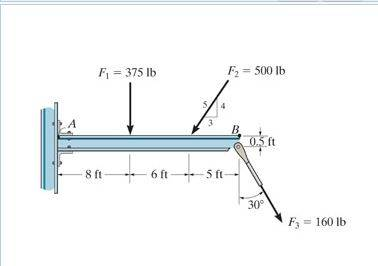# Statics: Finding the moment on point B?

## Homework Statement[/B]
Determine the moment about point B of each of the three forces acting on the beam.

M = F*d⊥

## The Attempt at a Solution

I went and found the components for each of the forces. Please double check it if I did them right:

F1 :
Fx = 0
Fy = 375 lb

F2:

Fx = -500(3/5)lb = -300lb
Fy = -500(4/5)lb = -400lb

F3:
Fx = 160cos30° = 138.6lb
Fy = -160sin30° = -80lb

If those components are right, I'm having trouble finding their perpendicular distances.

Based on the figure above is the
F*d⊥ for each of the forces are (without the sign convention/right hand method applied):
F1
Fx1 = 0
Fy1 = 375lb*19ft

F2
Fx2 = -300lb(0.5ft)
Fy2 = -400lb(19ft)

F3
Fx3 = 138.6lb(0.5ft)
Fx3 = -80lb(19ft)

I got 19ft by adding the three dimensions below the beam. Is that correct?

Label forces with F, and moments (force times distance) with M.
To calculate the moment of force F1 about point B, how far is F1 from point B?

Label forces with F, and moments (force times distance) with M.
To calculate the moment of force F1 about point B, how far is F1 from point B?
Ahh! 11 ft!